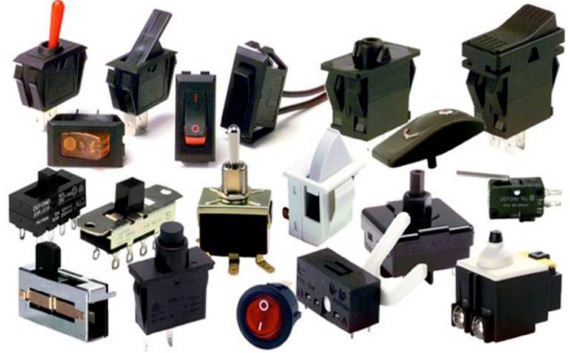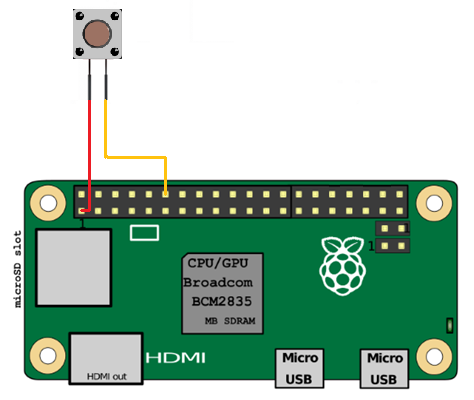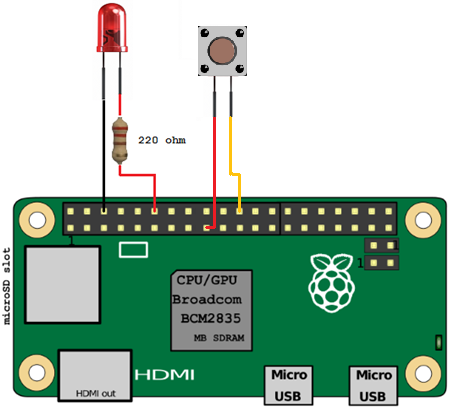# Raspberry Pi: Connecting a Switch

### Thursday 20th July 2017 1:35am

Switches come in numerous shapes and sizes. Some switches have latches and can be either switched on or switched off. Others are momentary, meaning they only operate when your finger presses them, as soon as you let go the switch is off.Collection of various switches

The switch can be wired to both the 3.3 volts (pin 1) and one of the GPIO pins; in this example I have chosen to use pin number 12 (refer to Connecting Hardware to a Raspberry Pi):Raspberry Pi Switch Wiring Diagram

To test the switch and wiring open a terminal window and type the following to start the Python interpreter:

```sudo python
```

Next type the following:

```import RPi.GPIO as GPIO
SWITCH_PIN = 12
GPIO.setmode(GPIO.BOARD)
GPIO.setup(SWITCH_PIN, GPIO.IN, GPIO.PUD_DOWN)
GPIO.setwarnings(False)

previous_state = False
current_state = False

while True:
previous_state = current_state
current_state = GPIO.input(SWITCH_PIN)
if current_state != previous_state:
new_state = "HIGH" if current_state else "LOW"
print("GPIO pin %s is %s" % (SWITCH_PIN, new_state))
```

If you press and hold down the switch the pin will go HIGH. Release the switch and the pin will go back to LOW:

```GPIO pin 12 is HIGH
GPIO pin 12 is LOW
GPIO pin 12 is HIGH
```

Press Ctrl + C when you want to exit.

## Using the switch to turn on an LED

We will now write a program to turn on an LED when the switch is pressed and turn off the LED when the switch is released. (Refer to Connecting an LED):Raspberry Pi Swtich and LED Wiring Diagram

```# Program to demonstrate connecting a switch
# that when pressed turns an LED on and when
# released turns the LED off.

import RPi.GPIO as GPIO

LED_PIN = 12
SWITCH_PIN = 22

GPIO.setmode(GPIO.BOARD)
GPIO.setmode(LED_PIN, GPIO.OUT)
GPIO.setup(SWITCH_PIN, GPIO.IN, GPIO.PUD_DOWN)
GPIO.setwarnings(False)

previous_state = False
current_state = True

while True:
previous_state = current_state
current_state = GPIO.input(SWITCH_PIN)
if current_state != previous_state:
new_state = “HIGH” if current_state else “LOW”
print(“GPIO pin %s is %s” % (SWITCH_PIN, new_state))

if new_state == “HIGH”:
GPIO.output(LED_PIN, GPIO.HIGH)
else:
GPIO.output(LED_PIN, GPIO.LOW)
```
FB Like & Share